# Command comparison: SM to ChIPS 4

Many astronomers use the excellent plotting program SM, by Robert Lupton and Patricia Monger (which had an interface derived in some respects from John Tonry's program Mongo). Here is a guide to using CIAO4's ChIPS for SM users.

We do not address contour or image plots or non-plotting SM commands in this document.

## Setup File

For the examples in this document to work as described, the following setup file must be loaded into the ChIPS session:

unix% cat chipsinit.py
set_preferences("axis.automin=false axis.automax=false line.style=solid curve.symbol.style=none")
set_preferences("foreground.display=black background.display=white")
add_plotarea( 0.0, 0.0, 1.0, 1.0, "id=global" )
points=ChipsCurve()
points.line.style="none"
points.symbol.style="cross"
points.symbol.size=4
points.symbol.color="black"
errors=ChipsCurve()
errors.line.style="none"
errors.symbol.style="none"
errors.err.style="cap"

unix% chips chipsinit.py
chips-1>


The first set_preferences command suppresses "nice" extra space around axes, so that the axis limits work precisely. The second set_preferences command sets the display colors: black foreground and white background. A plot area called "global" covers the whole frame so that we can easily work in "global" coordinates. Finally, ChIPS contexts called "points" and "errors" are defined; these are used use for plotting points in a style different from that used for plotting curves.

## Examples

### Example 1

SM Example ChIPS 4 Example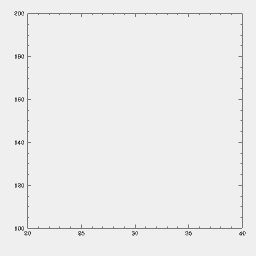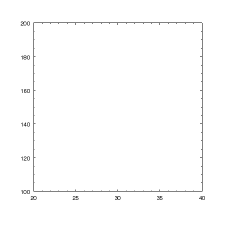limits 20 40 100 200
box

add_plotarea()
add_axis( X_AXIS, 0, 20, 40 )
add_axis( Y_AXIS, 0, 100, 200 )


The add_plotarea call sets things up for a new plot. It is needed before the add_axis commands on account of the global plot area defined in the setup file; normally it would be optional.

### Example 2

SM Example ChIPS 4 Example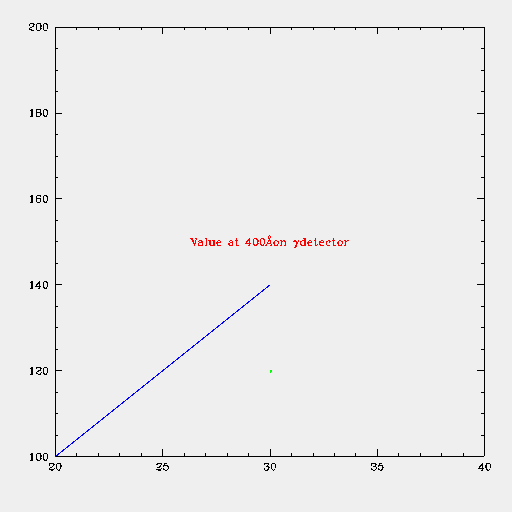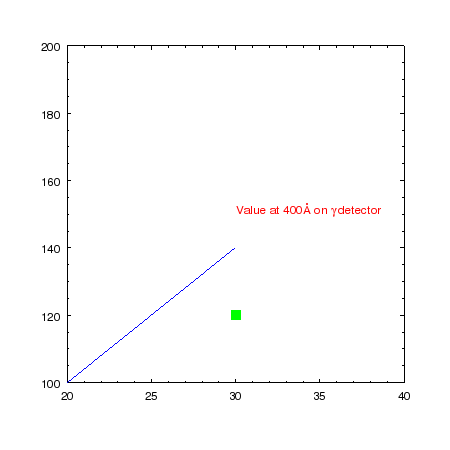limits 20 40 100 200
box
ctype green
relocate 30 120
ptype 4 3
dot
relocate 20 100
ctype blue
draw 30 140
ctype red
relocate 30 150
putlabel 5 "Value at 400\AA on \gamma detector"
ctype blue

add_plotarea()
add_axis( X_AXIS, 0, 20, 40 )
add_axis( Y_AXIS, 0, 100, 200 )
add_point( 30, 120, "color=green style=square" )
add_line( 20, 100, 30, 140, "color=blue" )
"Value at 400\\AA on \\gamma detector", "color=red" )


There are a number of different ways to define options; here the "name1=value1 name2=value2" approach is used. Other methods are described in the setting preferences documentation.

A variety of LaTeX escapes are available. Note that Latex commands in S-Lang must either use a double backslash or include the "r" modifier:

add_label( 30, 150, "Value at 400\\AA on \\gamma detector", "color=red" );

add_label( 30, 150, r"Value at 400\AA on \gamma detector", "color=red" );


### Example 3

SM Example ChIPS 4 Example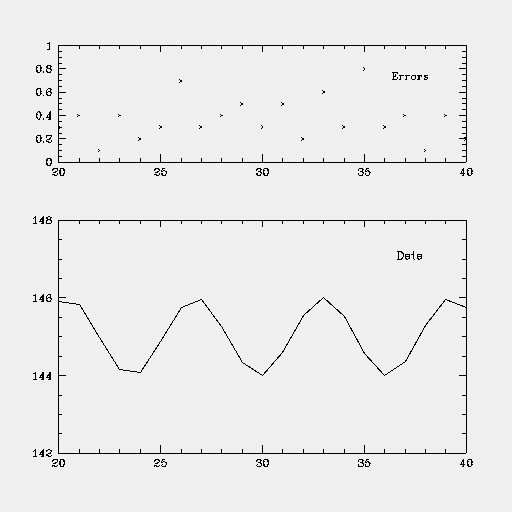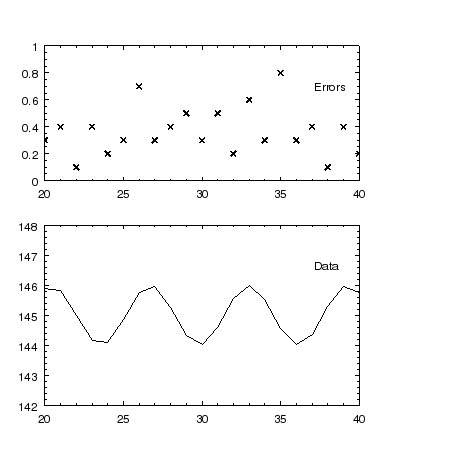define gx1 (int(37267*0.1))
define gx2 (int(37267*0.8))
define gy1 (int(37267*0.1))
define gy2 (int(37267*0.5))
define px1 (int(37267*0.1))
define px2 (int(37267*0.8))
define py1 (int(37267*0.6))
define py2 (int(37267*0.8))
data plot.dat
read { x 1 y 2 e 3 }
location $gx1$gx2 $gy1$gy2
limits 20 40 142 148
box
connect x y
location $px1$px2 $py1$py2
limits 20 40 0 1
box
points x e
location $gx1$gx2 $gy1$gy2
define glx (int(32767*0.8))
define gly (int(32767*0.5))
relocate ($glx$gly)
putlabel 5 Data
define glx (int(32767*0.8))
define gly (int(32767*0.85))
relocate ($glx$gly)
putlabel 5 Errors

set_plot( "style=open")
x = get_colvals( crate, 0 )
y = get_colvals( crate, 1 )
e = get_colvals( crate, 2 )
add_plotarea( 0.1, 0.1, 0.8, 0.5, "id=p1" )
add_axis( X_AXIS, 0, 20, 40 )
add_plotarea( 0.1, 0.6, 0.8, 0.9, "id=p2" )
add_axis( X_AXIS, 0, 20, 40 )
add_axis( Y_AXIS, 0, 0, 1 )
current_plot("global")


This is a more ambitious example which illustrates multiple plot areas and the use of global coordinates, as well as how to read columns from a file.

## Alphabetical List of Commands

The following is an alphabetical list of selected SM commands and the ChIPS equivalents.

SM Command SM Syntax ChIPS 4 Syntax
ANGLE
relocate x y
angle 45
dot
angle 0

relocate x y
angle 45   (for label)
putlabel 5 text
angle 0

add_point( x, y, "angle=45" )
add_label( x, y, text, "angle=45" )

AXIS
axis xmin xmax dx_small dx_big gx gy glen ilabel iclock
dx_small < 0  log  dx_small = 0 default
ilabel = 0  no labels
1  para axis
2 perp axis
3 no labels and no ticks

iclock = 0 1 ticks perp
2 3 ticks vert
4 5 ticks hor
(notation -8 8 -8 8)


ChIPS doesn't have a way to just draw a single axis with ticks, etc. The axis is tied to a coordinate system and drawn over the whole plot area.

For example, to draw a log X-axis with spacing 2 between the big ticks and 0.2 between the little ticks, starting at global coordinate gx, gy with length glen:

  gx2= gx + glen
add_plotarea( gx, gy, gx2, 1.0 )
add_axis( X_AXIS, 0, xmin, xmax, "majortick.interval=2.0
minortick.mode=interval minortick.interval=0.2
tickformat=%f" )
log_scale(X_AXIS)

BOX
box
box i1 i2 i3 i4


There is no equivalent command.

CONNECT
connect x y

add_curve( x, y )

CTYPE
ctype blue

....( ..., "color=blue" )
e.g.  add_line( x1, y1, x2, y2, "color = blue" )


add_curve( x, y, "line.color=blue" )

CURSOR
cursor x y

cursor = get_pick(0)

(terminate with ESC)
x = cursor
y = cursor

DATA
data myfile
read { x 1 y 3 z 12 }

file = read_file( "myfile" )
x = get_colvals( file, 0 )
y = get_colvals( file, 2 )
z = get_colvals( file, 11 )


Note that ChIPS is 0-based, so the columns are off by 1 from SM, and that you can also use the column name instead of the position in the get_colvals call.

DATE
label $date  Echoes date and time for plot label from time import ctime add_label( x, y, ctime())  DEV dev x11 dev postfile myfile.eps  ChIPS plots to an X11 window by default. It remembers the plot you made, and the print_window command can be used to create a postscript (or jpg, etc) file of the plot. If you just want to make a postscript file without displaying anything, switch off the ChIPS display: set_preference( "window.display","false" ) (... plotting commands ...) print_window( "myfile", "format=eps" )  DOT dot  add_point( x, y, "style=cross" )  DRAW relocate x1 y1 draw x2 y2  add_line( x1, y1, x2, y2 )  ERASE erase  erase()  ERRORBAR errorbar x y e iopt  The "errors" context defined in the setup file is used here. To add vertical error bars: add_curve( x, y, e, errors )  To plot horizontal error bars (with no vertical error bars) a list is passed to the command: add_curve( x, y, [None,None,e,e], errors )  The values "[None,None,e,e]" are the vectors for up, down, left and right errors respectively. EXPAND expand 3  A factor of 12 is about right to convert between SM and ChIPS sizes, so 36 (12*3) is used: add_point( x,y, "size=36") add_label( x, y, "My label text", "size=36" ) add_axis( x, y, ...., "label.size=36 ticklabel.size=36" )  FORMAT format xstring ystring  add_axis( ...., "tickformat=%f" )  SM accepts formats in Fortran or C style, but ChIPS accepts only C style: thus use "%6.2f" instead of "F6.2". GRID grid i j  add_axis( ..., "majorgrid.visible=true minorgrid.visible=true" )  HISTOGRAM hist x y  Draws in histogram style. (Do not confuse this with the get_hist macro which builds the histogram; this is a math operation which in ChIPS you'll have to do using the NumPy histogram function). add_histogram( x, y )  LABEL relocate x y label text  add_label( x, y, text )  LIMITS limits xmin xmax ymin ymax  The limits may be set when the axis is drawn: add_axis( X_AXIS, 0, xmin, xmax ) add_axis( Y_AXIS, 0, ymin, ymax )  The second parameter positions the axis. To change the limits after the axes are in place: limits( X_AXIS, xmin, xmax )  LOCATION If we define global coords x=0 to 1, y = 0 to 1, they have to be converted to 0-32767 in SM: define gx1 (int(32767*x1)) define gx2 (int(32767*x2)) define gy1 (int(32767*y1)) define gy2 (int(32767*y2)) location$gx1 $gx2$gy1 \$gy2

add_plotarea( gx1, gy1, gx2, gy2, "id=p1" )
...(plot commands)
current_plot("global")
... do something in global coords
current_plot("p2")
... do something in another plot area you've defined
current_plot("p1")
... back to the first plot area


Note the different order of the arguments. This defines a plot area p1; the current_plot call can be repeated to go back to that area after working in a different plot.

LTYPE
ltype 0

add_curve( x, y, "line.style=solid" )
add_line( x1, y1, x2, y2, "style=solid" )


The mapping between line types is

SM ChIPS style
0 1 Solid
1 2 Dot
2 6 Short dash
3 5 Long dash
4 4 Dot - short dash
5 3 Dot - long dash
6 7 Short dash - long dash
10 0 Erase lines
LWEIGHT
lweight 5

add_curve( x, y, "line.thickness=5")
add_line( x1, y1, x2, y2, "thickness=5" )

NOTATION
notation -8 8 -8 8


There is no direct equivalent; typical use cases can be handled by

add_axis( ...., "tickformat=%f")

POINTS
points x y


The "points" context defined in the setup file is used here. It is a set of options defining the size, color, and type of points and "turning off" the line which would connect them.

add_curve( x, y, points)

PTYPE
ptype 4 3

add_point( ..., "style=square fill=true" )


or

points.symbol.style="square"
points.symbol.fill="true"


Note that "ptype x", where x is a vector, is not supported in ChIPS.

The point styles are:

SM ChIPS option Style
1 1 style=point dot
3 0 style=uptriangle open triangle
3 3 style=uptriangle fill=true solid triangle
4 0 style=square Open square
4 1 style=plus Plus
4 1 (angle=45) style=cross Cross
4 3 style=square fill=true Filled square
10 0 style=circle Open circle

Pentagon and hexagon are not supported.

PUTLABEL

see LABEL

QUIT
quit
quit

see DATA

RELOCATE
relocate x y
dot
relocate x y
draw x2 y2

add_point( x, y )
add_line( x1, y1, x2, y2 )

shade n x y

add_region( ... ,